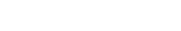VISION1 Tensorflow模型文件

|--checkpoint_dir
|    |--checkpoint
|    |--MyModel.meta
|    |--MyModel.data-00000-of-00001
|    |--MyModel.index

1.1 meta文件

MyModel.meta文件保存的是图结构，meta文件是pb（protocol buffer）格式文件，包含变量、op、集合等。

1.2 ckpt文件

MyModel.data-00000-of-00001
MyModel.index

2 保存Tensorflow模型

tensorflow 提供了tf.train.Saver类来保存模型，值得注意的是，在tensorflow中，变量是存在于Session环境中，也就是说，只有在Session环境下才会存有变量值，因此，保存模型时需要传入session：

saver = tf.train.Saver()
saver.save(sess,"./checkpoint_dir/MyModel")

import tensorflow as tf

w1 = tf.Variable(tf.random_normal(shape=), name='w1')
w2 = tf.Variable(tf.random_normal(shape=), name='w2')
saver = tf.train.Saver()
sess = tf.Session()
sess.run(tf.global_variables_initializer())
saver.save(sess, './checkpoint_dir/MyModel')

checkpoint
MyModel.data-00000-of-00001
MyModel.index
MyModel.meta

saver.save(sess, './checkpoint_dir/MyModel',global_step=1000)

checkpoint
MyModel-1000.data-00000-of-00001
MyModel-1000.index
MyModel-1000.meta

saver.save(sess, './checkpoint_dir/MyModel',global_step=step,write_meta_graph=False)

tf.train.Saver(max_to_keep=5, keep_checkpoint_every_n_hours=2)

import tensorflow as tf
w1 = tf.Variable(tf.random_normal(shape=), name='w1')
w2 = tf.Variable(tf.random_normal(shape=), name='w2')
saver = tf.train.Saver([w1,w2])
sess = tf.Session()
sess.run(tf.global_variables_initializer())
saver.save(sess, './checkpoint_dir/MyModel',global_step=1000)

3 导入训练好的模型

3.1 构造网络图

saver=tf.train.import_meta_graph('./checkpoint_dir/MyModel-1000.meta')

3.2 加载参数

import tensorflow as tf
with tf.Session() as sess:
new_saver = tf.train.import_meta_graph('./checkpoint_dir/MyModel-1000.meta')
new_saver.restore(sess, tf.train.latest_checkpoint('./checkpoint_dir'))

import tensorflow as tf
with tf.Session() as sess:
saver = tf.train.import_meta_graph('./checkpoint_dir/MyModel-1000.meta')
saver.restore(sess,tf.train.latest_checkpoint('./checkpoint_dir'))
print(sess.run('w1:0'))
##Model has been restored. Above statement will print the saved value

[ 0.51480412 -0.56989086]

4 使用恢复的模型

import tensorflow as tf

w1 = tf.placeholder("float", name="w1")
w2 = tf.placeholder("float", name="w2")
b1= tf.Variable(2.0,name="bias")

#定义一个op，用于后面恢复
w4 = tf.multiply(w3,b1,name="op_to_restore")
sess = tf.Session()
sess.run(tf.global_variables_initializer())

#创建一个Saver对象，用于保存所有变量
saver = tf.train.Saver()

#通过传入数据，执行op
print(sess.run(w4,feed_dict ={w1:4,w2:8}))
#打印 24.0 ==>(w1+w2)*b1

#现在保存模型
saver.save(sess, './checkpoint_dir/MyModel',global_step=1000)

import tensorflow as tf

sess=tf.Session()
#先加载图和参数变量
saver = tf.train.import_meta_graph('./checkpoint_dir/MyModel-1000.meta')
saver.restore(sess, tf.train.latest_checkpoint('./checkpoint_dir'))

# 访问placeholders变量，并且创建feed-dict来作为placeholders的新值
graph = tf.get_default_graph()
w1 = graph.get_tensor_by_name("w1:0")
w2 = graph.get_tensor_by_name("w2:0")
feed_dict ={w1:13.0,w2:17.0}

#接下来，访问你想要执行的op
op_to_restore = graph.get_tensor_by_name("op_to_restore:0")

print(sess.run(op_to_restore,feed_dict))
#打印结果为60.0==>(13+17)*2

import tensorflow as tf

sess = tf.Session()
# 先加载图和变量
saver = tf.train.import_meta_graph('my_test_model-1000.meta')
saver.restore(sess, tf.train.latest_checkpoint('./'))

# 访问placeholders变量，并且创建feed-dict来作为placeholders的新值
graph = tf.get_default_graph()
w1 = graph.get_tensor_by_name("w1:0")
w2 = graph.get_tensor_by_name("w2:0")
feed_dict = {w1: 13.0, w2: 17.0}

#接下来，访问你想要执行的op
op_to_restore = graph.get_tensor_by_name("op_to_restore:0")

# 在当前图中能够加入op

# 打印120.0==>(13+17)*2*2

......
......
saver = tf.train.import_meta_graph('vgg.meta')
# 访问图
graph = tf.get_default_graph()

#访问用于fine-tuning的output
fc7= graph.get_tensor_by_name('fc7:0')

#如果你想修改最后一层梯度，需要如下
fc7 = tf.stop_gradient(fc7) # It's an identity function
fc7_shape= fc7.get_shape().as_list()

new_outputs=2
weights = tf.Variable(tf.truncated_normal([fc7_shape, num_outputs], stddev=0.05))
biases = tf.Variable(tf.constant(0.05, shape=[num_outputs]))
output = tf.matmul(fc7, weights) + biases
pred = tf.nn.softmax(output)

# Now, you run this with fine-tuning data in sess.run()• 唐山赫鸣科技有限公司 冀ICP备11004205号-1